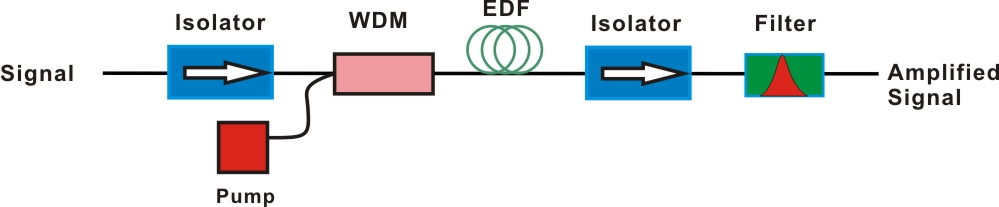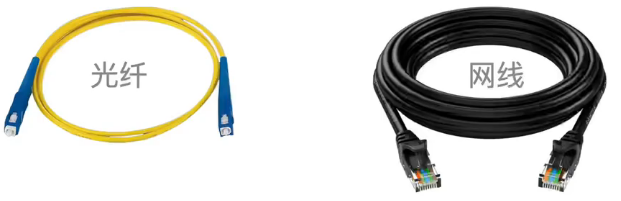## EDFAEDFA能放大的波长：$$1.53 \sim 1.56 \mu \mathrm{m}$$，其中$$1.55 \mu \mathrm{m}$$的损耗最低。

## dB

dB分贝(decibel)是量度两个相同单位之数量比例的单位，常用$$\mathrm{dB}$$表示。deci表示十分之一(注：decimal representation小数表示，decimal，十进制的，十进制，小数m，十分之一的)；bel是为了纪念发明家贝尔(注：就是贝尔实验室、贝尔电话公司)。

$$\mathrm{dB}$$就是十分之一$$\mathrm{B}$$(贝)。$$1 \mathrm{~B}=10 \mathrm{~dB}$$。可分为两种情况：

• $$1$$贝尔的两个功率量的比值是$$10: 1$$。场量(field quantity)是诸如电压、电流、声压、电场强度、速度、电荷密度等量值，其平方值在一个线性系统中与功率成比例。考虑到场(field)的幅值(amplitude)时，通常使用$$A_1$$(度量到的幅值)的平方与$$A_0$$(参考幅值)的平方之比。这是因为对于大多数应用，功率与幅值的平方成比例，并期望对同一应用采取功率计算的分贝与用场的幅值计算的分贝相等。因此使用下述场量的分贝定义：$$L_{\mathrm{dB}}=10 \log _{10}\left(\frac{A_1^2}{A_0^2}\right)=20 \log _{10}\left(\frac{A_1}{A_0}\right)$$
• $$1$$贝尔的两个场量的比值是$$\sqrt{10}: 1$$。功率量(power quantity)是功率值或者直接与功率值成比例的其它量，如能量密度、音强、发光强度等。 分贝的计算，依赖于是功率量还是场量而不同。考虑功率或者强度(intensity)时，其比值可以表示为分贝，这是通过把测量值与参考量值之比计算基于$$10$$的对数，再乘以$$10$$。因此功率值$$P_1$$与另一个功率值$$P_0$$之比用分贝表示为$$L_{\mathrm{dB}}$$$$L_{\mathrm{dB}}=10 \log _{10}\left(\frac{P_1}{P_0}\right)$$

• 分贝实际上是对数值，因此可以用常用的数量来表示非常大的比值，可以清楚地表示非常大的数量变化。
• 多部件系统的整体增益(如级联的放大器)可以直接用各部件的增益分贝相加而求得。不必把这些增益值相乘(例如$$\log (A \times B \times C)=\log (A)+\log (B)+\log (C)$$)。
• 人对强度的感知，如声音或者光照，更接近与强度的对数成正比而不是强度值本身，依据韦伯-费希纳定律，因此分贝值可用于描述感知级别或级差。

• 声音—声学中，声音的强度定义为声压。其中$$p_{\text {ref }}$$人类对声音能够感知的阈值下限，$$L_p=20 \log _{10}\left(\frac{p_{\text {rms }}}{p_{\text {ref }}}\right) \mathrm{dB}$$
• 光学—
• 光谱学中吸光度的单位是$$-1 B$$，吸光度定义为$$A_\lambda=\log _{10}\left(I_0 / I_1\right)=-\log _{10}(\mathcal{T})$$，其中$$\mathcal{T}$$为透光率；
• 在光路中，如果已知功率的光注入光纤，每个电子元件(例如连接器、接头器、光纤长度)损失的分贝值也是已知的，整个光路损失可以通过加、减分贝值而快速求得
• 电子学—通常用分贝表示功率或幅值之比(增益)，而不常用算术比或者百分比。一项好处是一些列部件组成的系统的总增益是各部件增益之和。类似的，电信领域中，从一个发射器到一个接收器通过一些媒介(光无线通信、波导、同轴电缆、光纤等等)的信号增益用分贝表示，用于链路预算。

• $$+3 \mathrm{~dB}$$是指增加为$$2$$倍大；
• $$+10 \mathrm{~dB}$$是指增加为$$10$$倍大；
• $$-3 \mathrm{~dB}$$是指减小到$$1/2$$；
• $$-10 \mathrm{~dB}$$是指减小到$$1/10$$；

xx

Weber states that, "the minimum increase of stimulus which will produce a perceptible increase of sensation is proportional to the pre-existent stimulus," while Fechner's law is an inference from Weber's law (with additional assumptions) which states that the intensity of our sensation increases as the logarithm of an increase in energy rather than as rapidly as the increase.

• 例子-1：左手举300克砝码，左手举305克砝码，他并不会感觉差别有多少，直到左手重量加到306克砝码时，才觉得有些重；如果右手举600克砝码，这时，左手重量要达到612克才感觉到重。 也就是说，原来的砝码越重，后来必须加更大量才能感觉到差别，这种现象被称为，“韦伯-费希纳定律(Weber-FechnerLaw) ”，也叫“感觉适应”。
• 例子-2：你在一个嘈杂环境中，必须大声吼出来，别人才能听到你说话；而在一个安静房间里，细声细语也能被听到。
• 例子-3：因为恋爱时间的延长，对方做出相同的爱情行动，你的感觉越来越弱。

• 阈限：是物理刺激能量可以被个人觉察的临界点。
• 绝对阈限：是个体对单一刺激引起感觉经验时，所需最低的刺激强度，分为上绝对阈及下绝对阈。
• 差异阈限：又称恰辨差，指辨别两个刺激之间的差异时，这两种刺激强度最低的差异量。

The perceived change in stimuli is proportional to the initial stimuli.比如$$d p=\displaystyle\frac{d S}{S}$$，其中$$dS$$是实际的变化量，而$$dp$$是你感知到的变化量。进一步地，Perceived loudness/brightness is proportional to logarithm of the actual intensity measured with an accurate nonhuman instrument. 数学公式可以表示为$$p=k \ln \displaystyle\frac{S}{S_0}$$

(1) dB意味着：简单！所以，你不可以不明白！—知乎
(2) 分贝—Wiki

https://blog.csdn.net/qq_38938670/article/details/108348362

https://www.rp-photonics.com/passive_fiber_optics.html

## 小知识

### 光纤和网线## 光子晶体光纤

http://www.cailiaoniu.com/38123.html

http://www.phomera.com/zp

https://www.zhihu.com/question/25983978

http://www.nanojanus.com/page.php?mod=detail&id=1&cateid=1

http://www.nanojanus.com/article.php?mod=detail&id=6&cid=1&parentid=0

https://www.sohu.com/a/324056823_120118831

https://slidesplayer.com/slide/16054308/

https://cn.comsol.com/blogs/engineering-the-flow-of-light-using-photonic-crystals/